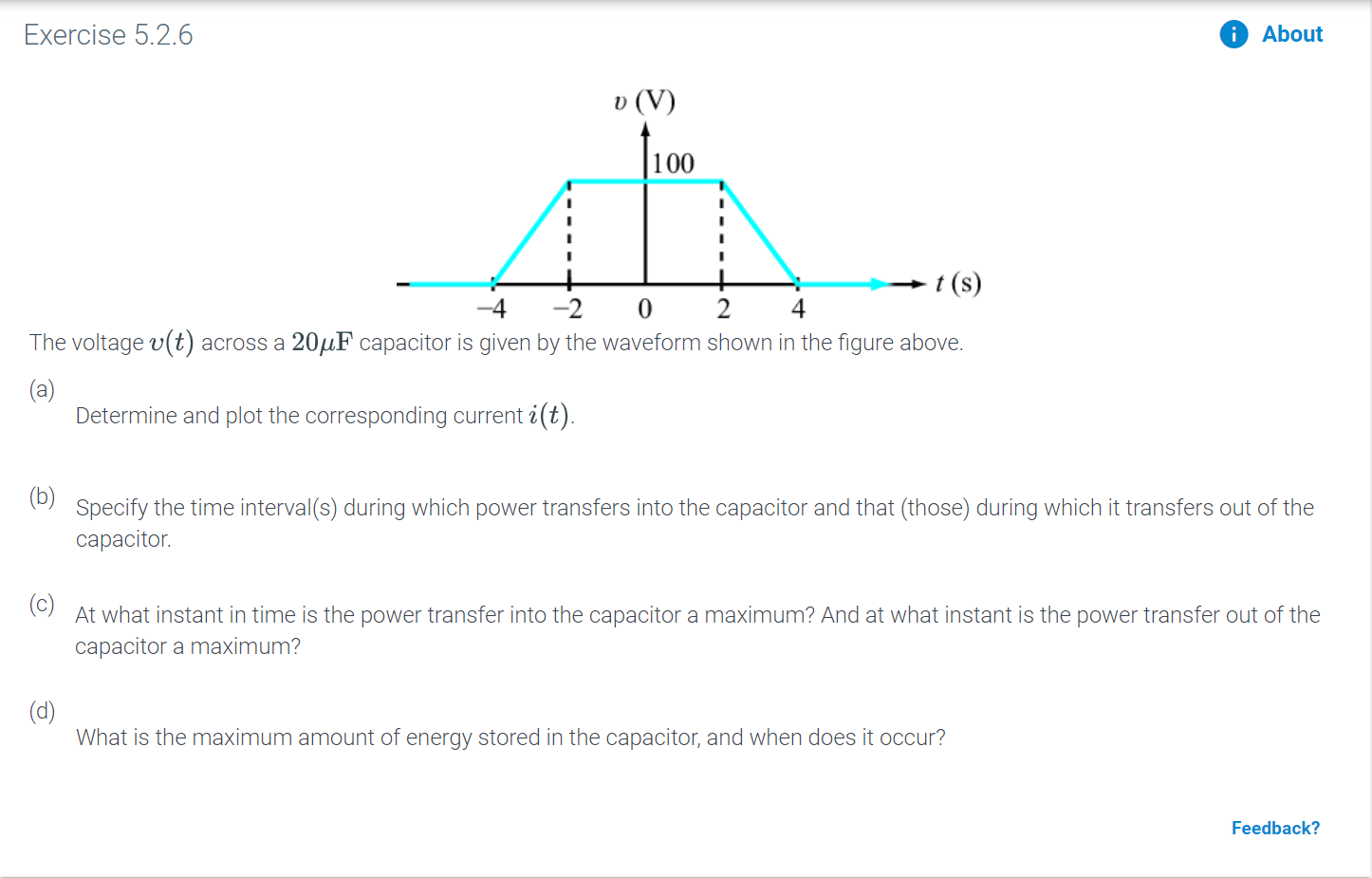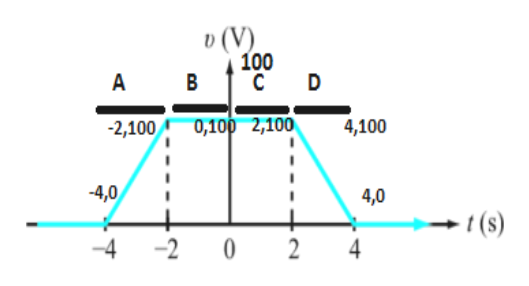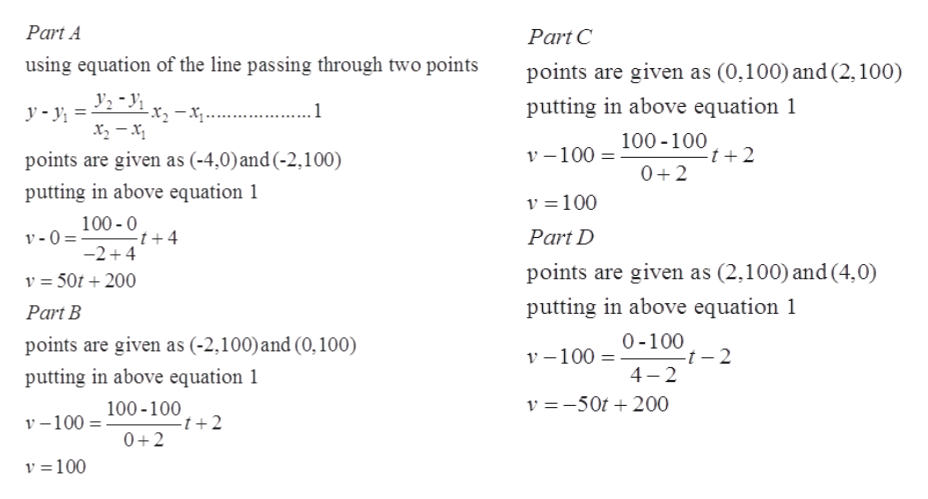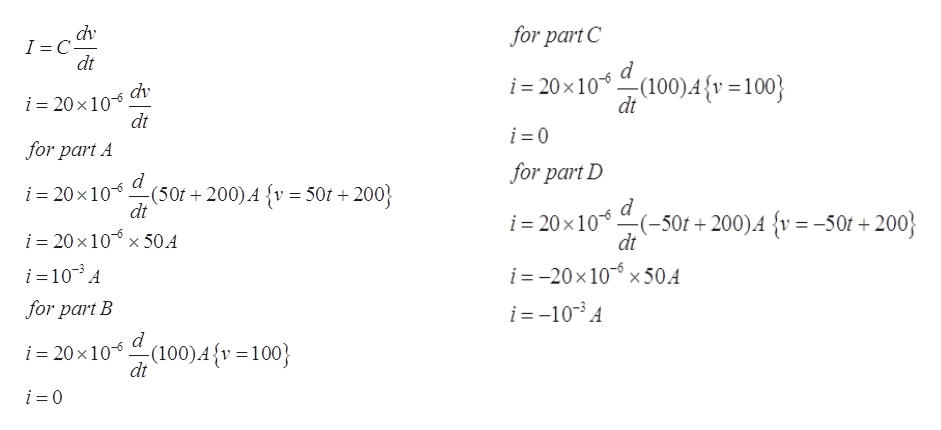# Exercise 5.2.6Aboutv (V100It(s)4-2024The voltage v(t) across a 204F capacitor is given by the waveform shown in the figure above.(а)Determine and plot the corresponding current i(t).Specify the time interval(s) during which power transfers into the capacitor and that (those) during which it transfers out of theсарacitor.(c)At what instant in time is the power transfer into the capacitor a maximum? And at what instant is the power transfer out of thecapacitor a maximum?(d)What is the maximum amount of energy stored in the capacitor, and when does it occur?Feedback?

Question
41 viewshelp_outlineImage TranscriptioncloseExercise 5.2.6 About v (V 100 I t(s) 4 -2 0 2 4 The voltage v(t) across a 204F capacitor is given by the waveform shown in the figure above. (а) Determine and plot the corresponding current i(t). Specify the time interval(s) during which power transfers into the capacitor and that (those) during which it transfers out of the сарacitor. (c) At what instant in time is the power transfer into the capacitor a maximum? And at what instant is the power transfer out of the capacitor a maximum? (d) What is the maximum amount of energy stored in the capacitor, and when does it occur? Feedback? fullscreen
check_circle

Step 1

As per our honor code  we can only answer up to 3 sub-parts, we’ll answer the first 3. Please resubmit the question and specify the other subparts (up to 3) you would  like answered.

(a)The graph of voltage versus time of the capacitor is shown below. The given graph can be split into four-time ranges as shown below.help_outlineImage Transcriptionclose(V) 100 В A C 4,100 0,100 2,100 -2,100 -4,0 4,0 t (s) -4 2 0 2 fullscreen
Step 2

Evaluation of equations for the given four regions A,B,C and D. The equations for the different regions A,B,C and D is shown below:help_outlineImage TranscriptionclosePart A Part C using equation of the line passing through two points points are given as (0,100) and (2,100) -x. putting in above equation 1 y-y 100-100 v-100 points are given as (-4,0) and (-2,100) 0+2 putting in above equation 1 v 100 100-0 t +4 -2+4 Part D v-0= points are given as (2,100) and (4,0) v 50t200 putting in above equation 1 Part B 0-100 -t - 2 4 2 points are given as (-2,100) and (0, 100) v-100 putting in above equation 1 v -50t200 100-100 -t + 2 v-100 0+2 v 100 fullscreen
Step 3

Calculation of the current for the given regions A,B,C and D. The equation of the current ...help_outlineImage Transcriptionclosedv I C dt for part C d i 20x10 dt (100)A {v = 100} dv i = 20 x 10 dt i 0 for part A for part D d i = 20x 10(50t 200) A {v = 50t +200 dt i 20x10 dt (-50f+ 200)A {v= -50r+200 20 x 10 i x 50.4 i =-20x 10x50.A i =-103A i =103A for part B d i =20 x10(100),A {v =100} dt i 0 fullscreen

### Want to see the full answer?

See Solution

#### Want to see this answer and more?

Solutions are written by subject experts who are available 24/7. Questions are typically answered within 1 hour.*

See Solution
*Response times may vary by subject and question.
Tagged in

### Electrical Engineering